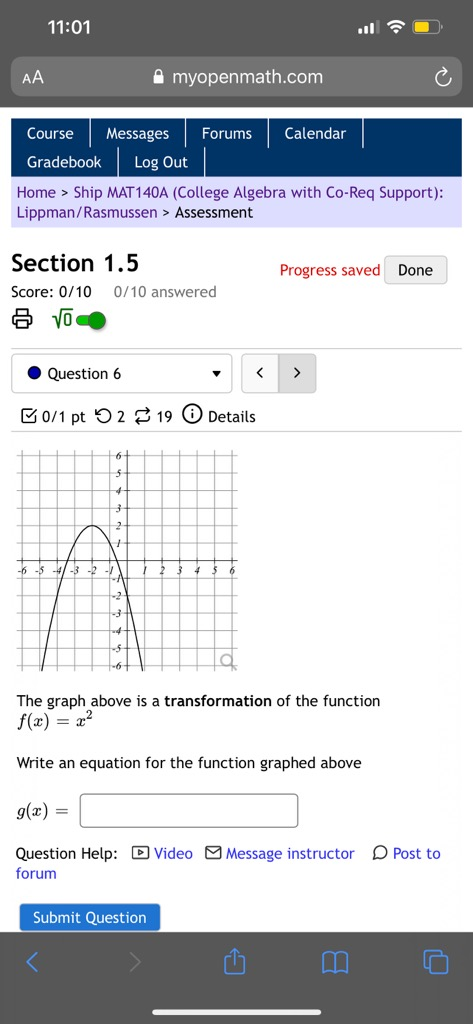# -6 -5 4-3 -2 The graph above is a transformation of the function f(x) = 2? Write an equation for the function graphed above g(x) : Question Help: D | Video Message instructor D Po forum

Question

The graph above is the transformation of the function f(x)=x^2help_outlineImage Transcriptionclose-6 -5 4-3 -2 The graph above is a transformation of the function f(x) = 2? Write an equation for the function graphed above g(x) : Question Help: D | Video Message instructor D Po forum fullscreen

### Want to see this answer and more?

Experts are waiting 24/7 to provide step-by-step solutions in as fast as 30 minutes!*

*Response times may vary by subject and question complexity. Median response time is 34 minutes for paid subscribers and may be longer for promotional offers.
Tagged in
Math
Algebra

### Equations and In-equations